# RS Aggarwal Solutions for Class 7 Maths Exercise 20G Chapter 20 Mensuration

RS Aggarwal Solutions for Class 7 Maths Exercise 20G Chapter 20 Mensuration in simple PDF are given here. This exercise of RS Aggarwal Solutions for Class 7 Chapter 20 contains objective type questions. It covers all the topics in this chapter. Students can learn more about these topics by solving the problems of RS Aggarwal Solutions for Class 7 Maths Chapter 20 Mensuration Exercise 20G with the help of solutions provided here.

## Download the PDF of RS Aggarwal Solutions For Class 7 Maths Chapter 20 Mensuration – Exercise 20G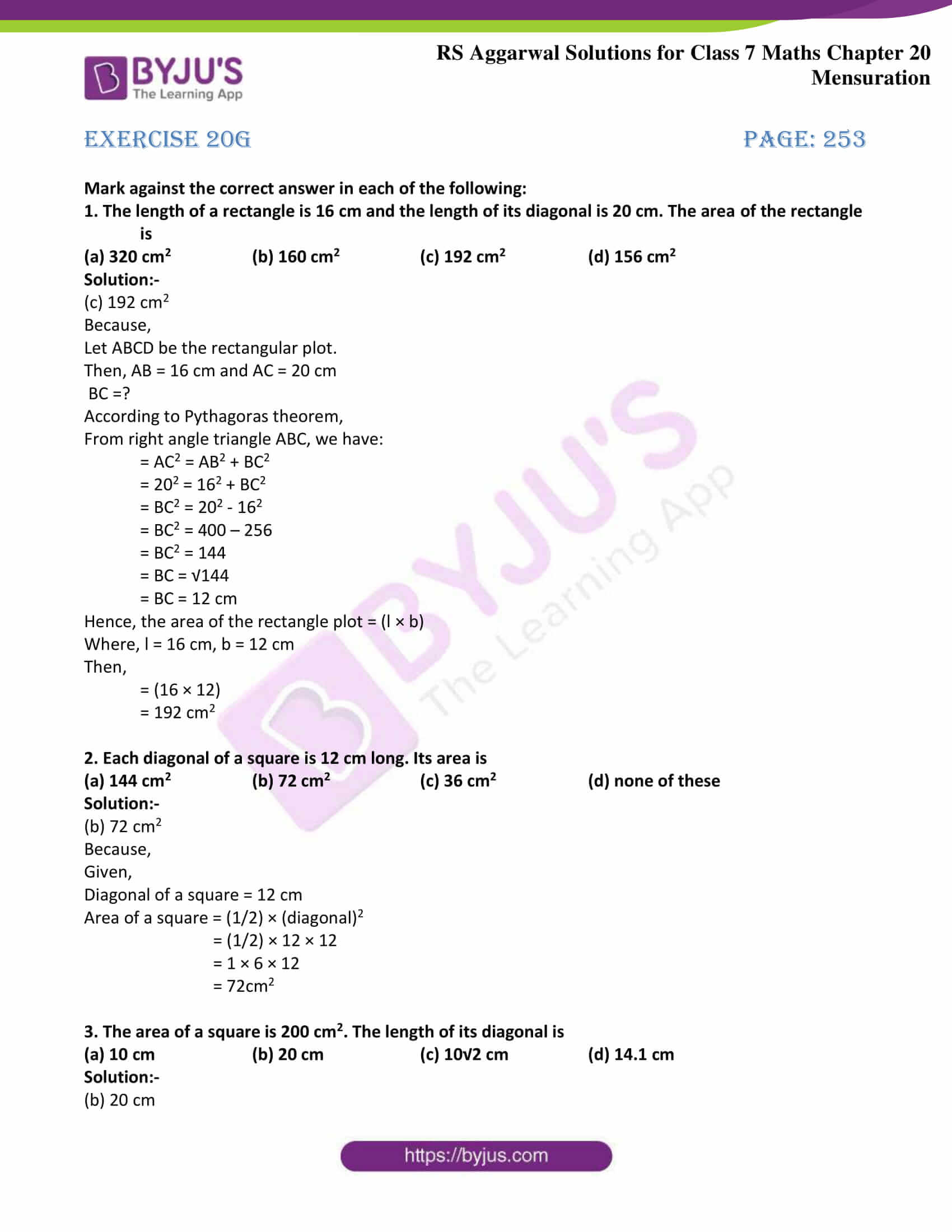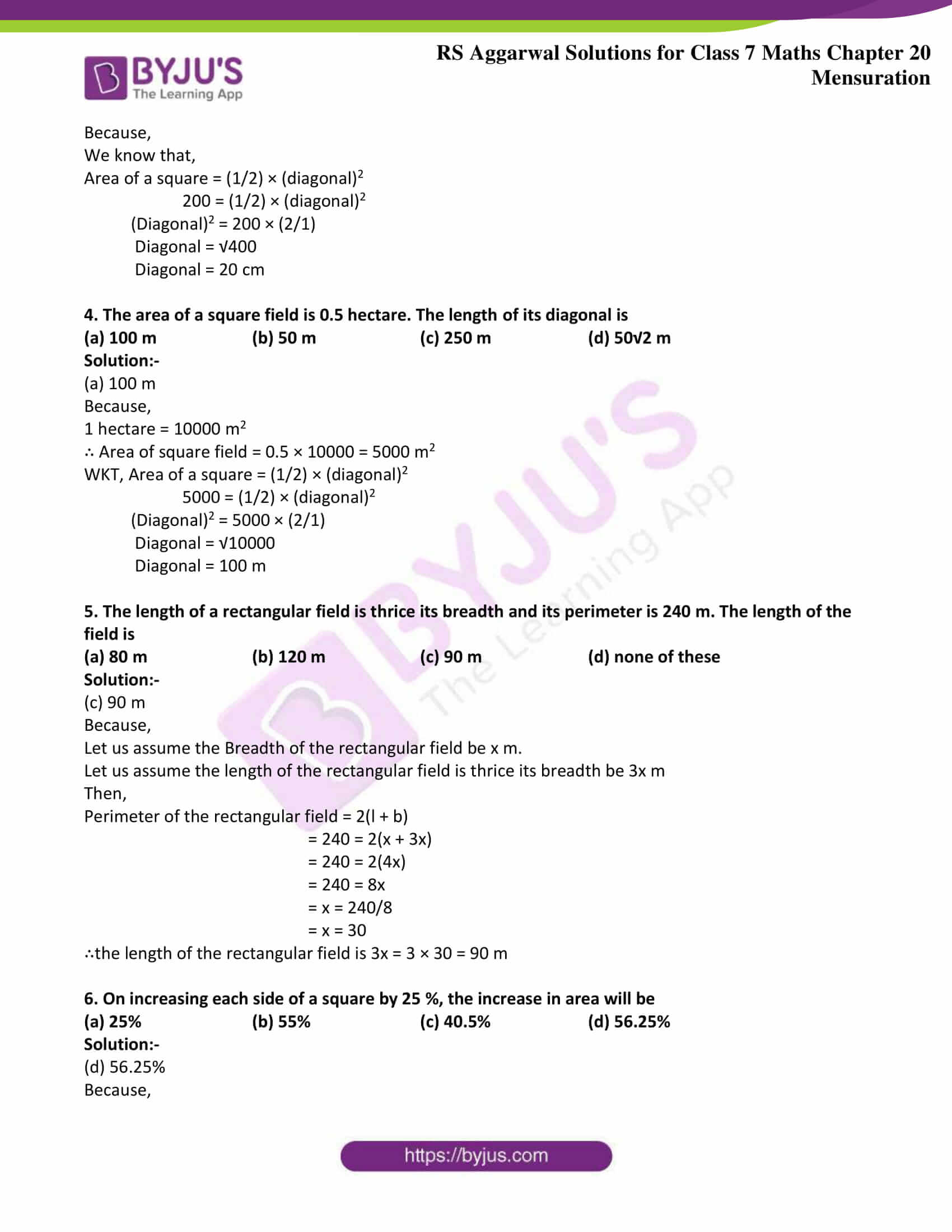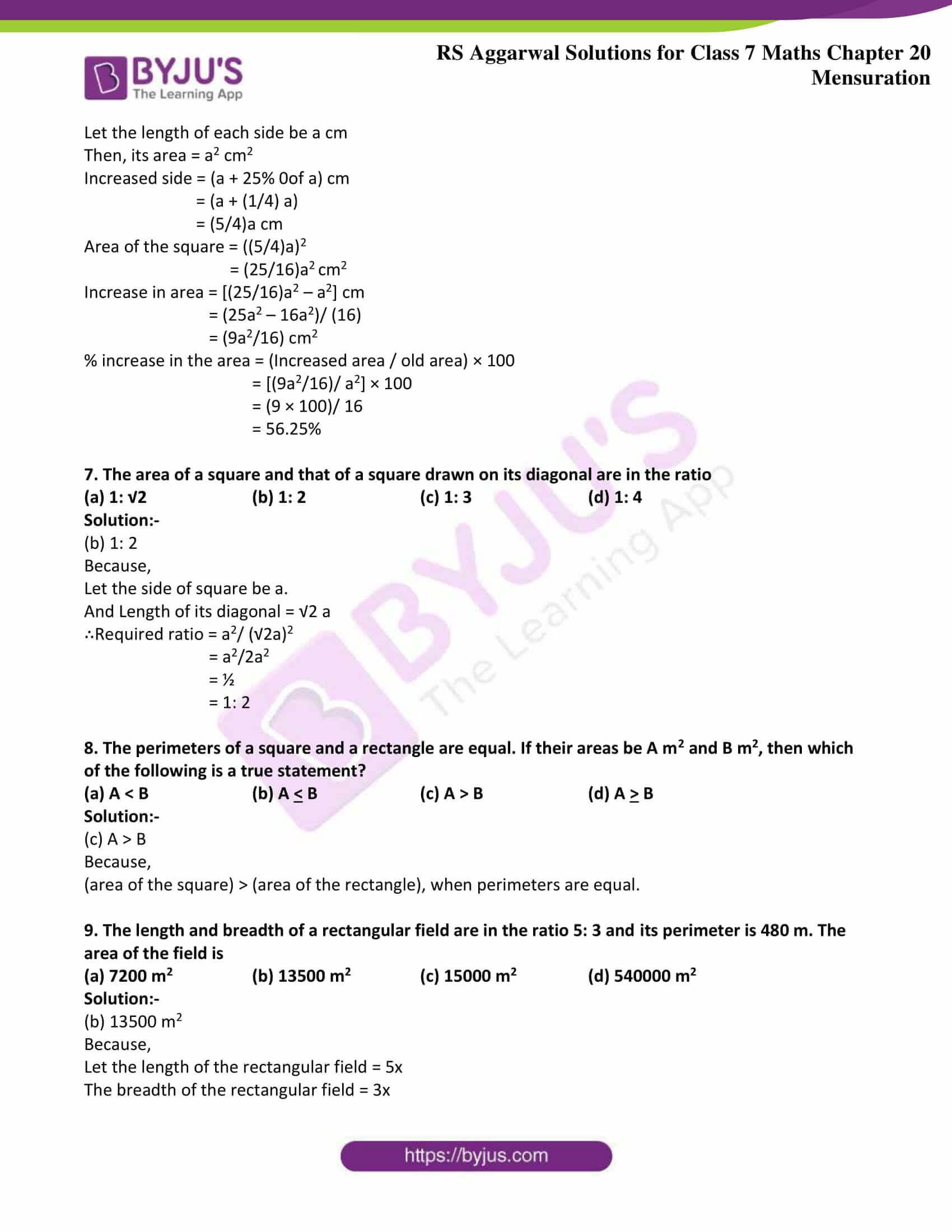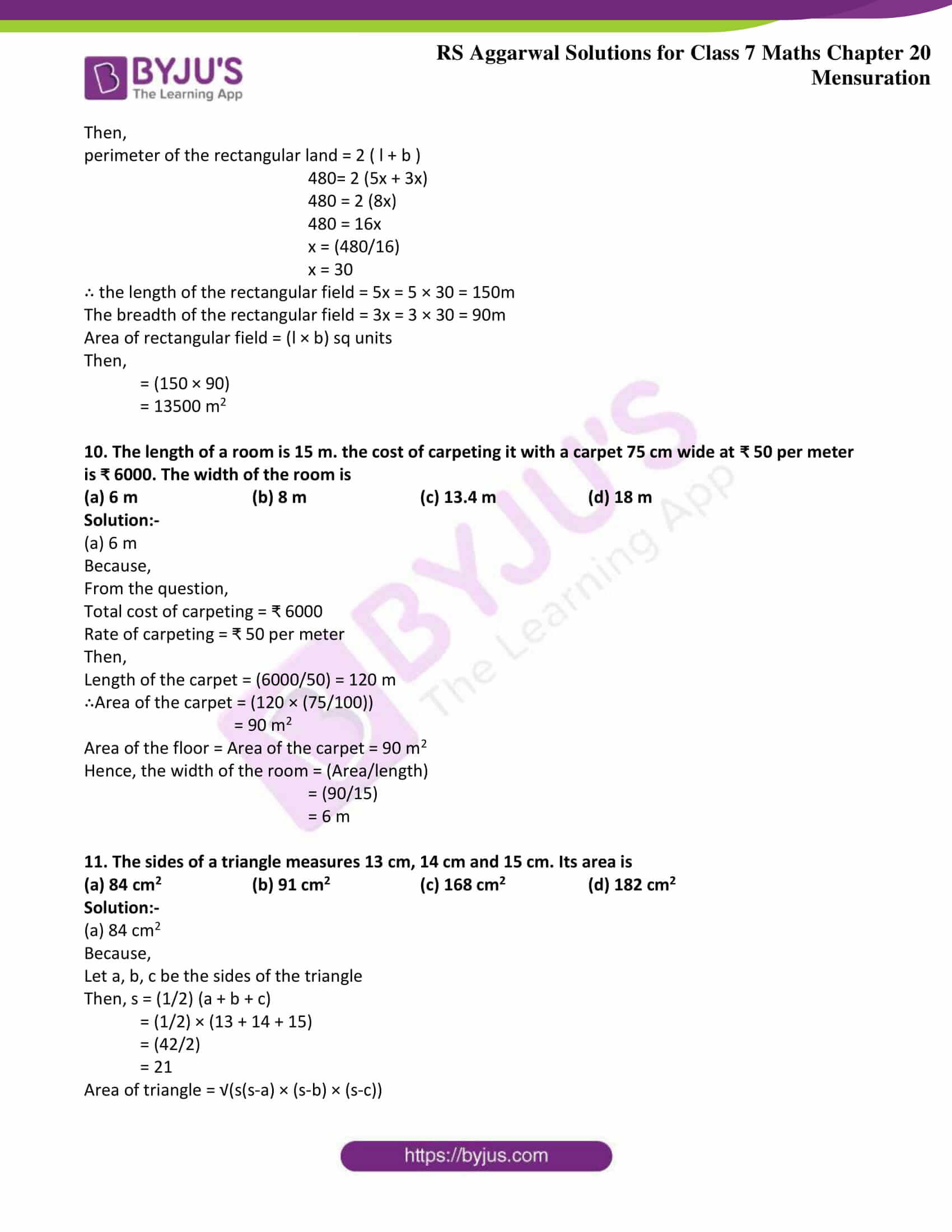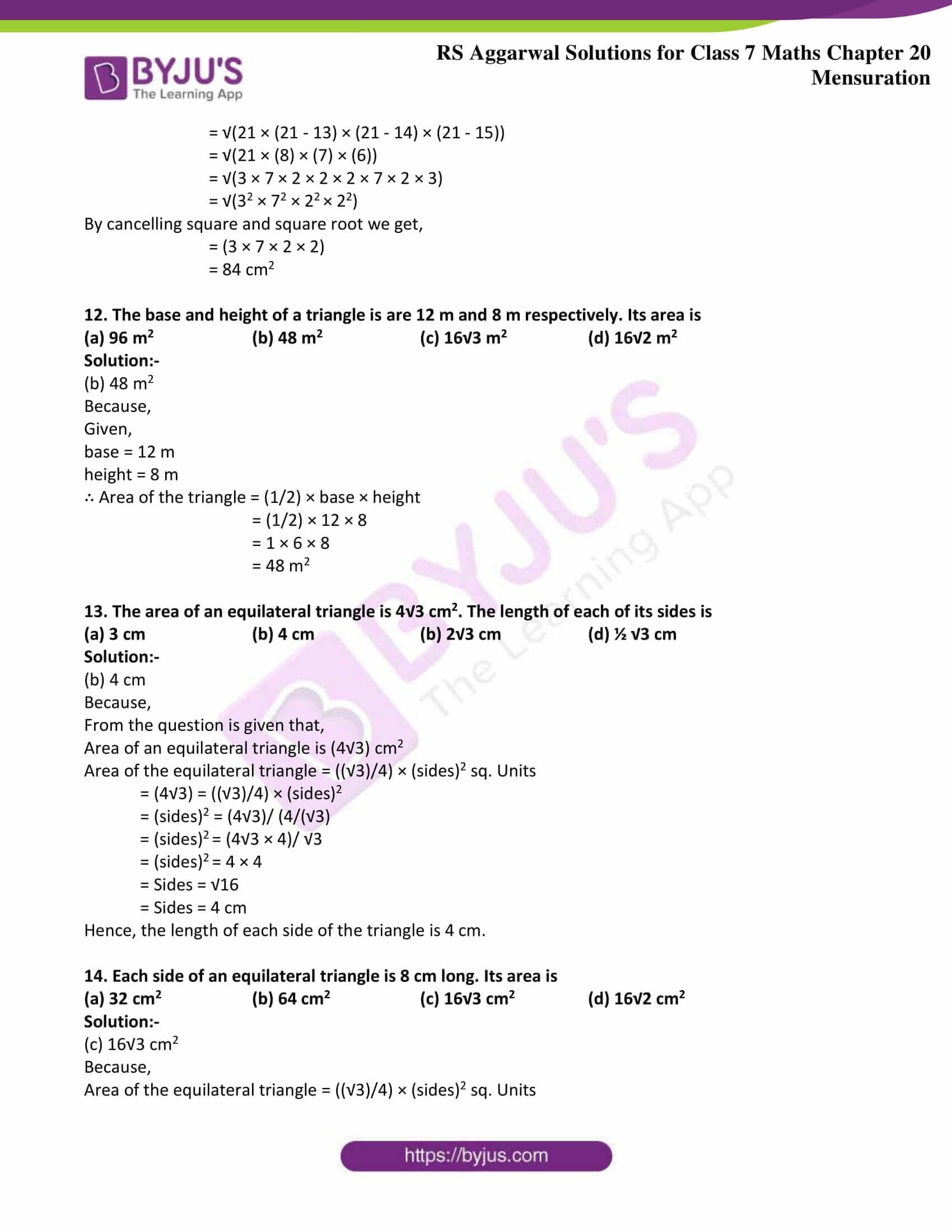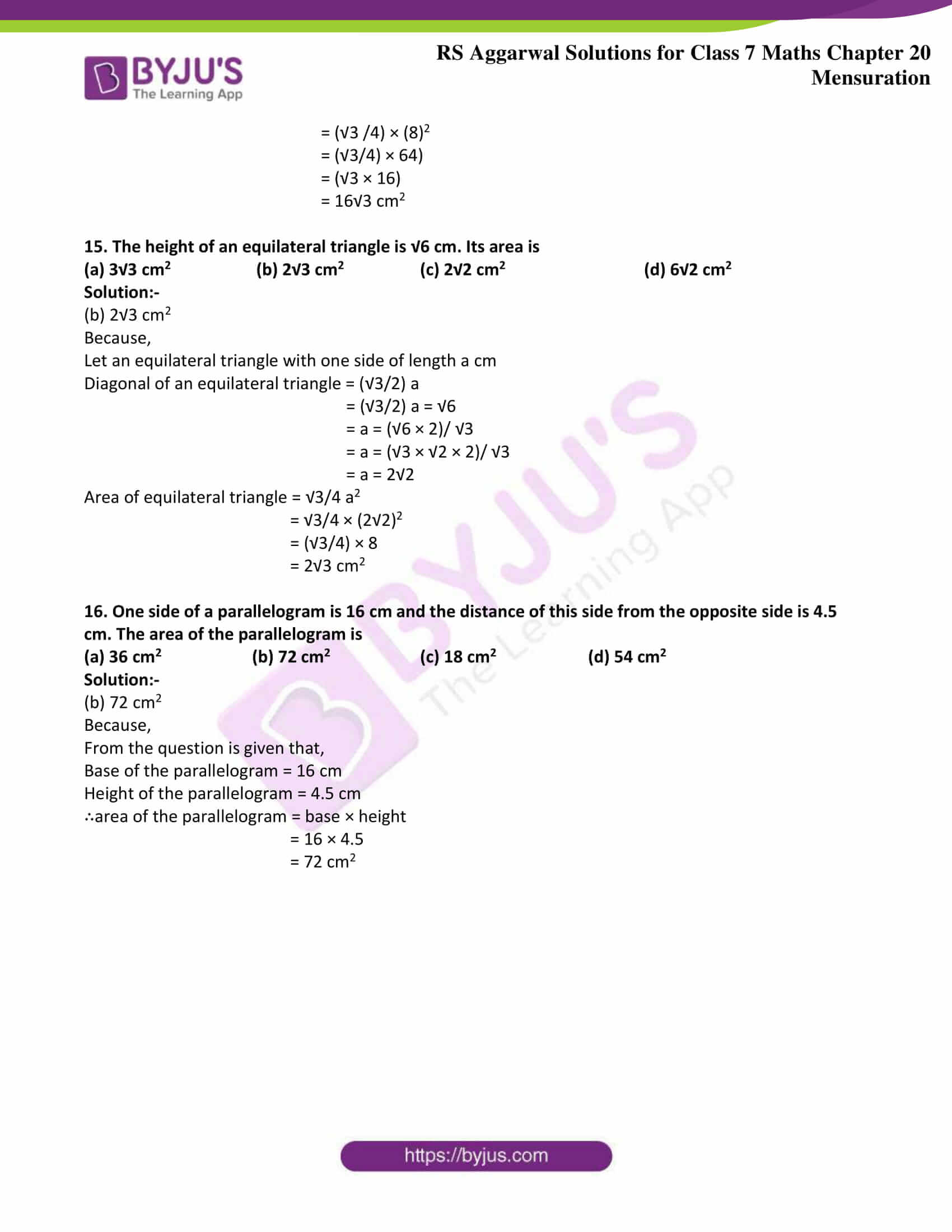### Access answers to Maths RS Aggarwal Solutions for Class 7 Chapter 20 – Mensuration Exercise 20G

Mark against the correct answer in each of the following:

1. The length of a rectangle is 16 cm and the length of its diagonal is 20 cm. The area of the rectangle is

(a) 320 cm2 (b) 160 cm2 (c) 192 cm2 (d) 156 cm2

Solution:-

(c) 192 cm2

Because,

Let ABCD be the rectangular plot.

Then, AB = 16 cm and AC = 20 cm

BC =?

According to Pythagoras theorem,

From right angle triangle ABC, we have:

= AC2 = AB2 + BC2

= 202 = 162 + BC2

= BC2 = 202 – 162

= BC2 = 400 – 256

= BC2 = 144

= BC = √144

= BC = 12 cm

Hence, the area of the rectangle plot = (l × b)

Where, l = 16 cm, b = 12 cm

Then,

= (16 × 12)

= 192 cm2

2. Each diagonal of a square is 12 cm long. Its area is

(a) 144 cm2 (b) 72 cm2 (c) 36 cm2 (d) none of these

Solution:-

(b) 72 cm2

Because,

Given,

Diagonal of a square = 12 cm

Area of a square = (1/2) × (diagonal)2

= (1/2) × 12 × 12

= 1 × 6 × 12

= 72cm2

3. The area of a square is 200 cm2. The length of its diagonal is

(a) 10 cm (b) 20 cm (c) 10√2 cm (d) 14.1 cm

Solution:-

(b) 20 cm

Because,

We know that,

Area of a square = (1/2) × (diagonal)2

200 = (1/2) × (diagonal)2

(Diagonal)2 = 200 × (2/1)

Diagonal = √400

Diagonal = 20 cm

4. The area of a square field is 0.5 hectare. The length of its diagonal is

(a) 100 m (b) 50 m (c) 250 m (d) 50√2 m

Solution:-

(a) 100 m

Because,

1 hectare = 10000 m2

∴ Area of square field = 0.5 × 10000 = 5000 m2

WKT, Area of a square = (1/2) × (diagonal)2

5000 = (1/2) × (diagonal)2

(Diagonal)2 = 5000 × (2/1)

Diagonal = √10000

Diagonal = 100 m

5. The length of a rectangular field is thrice its breadth and its perimeter is 240 m. The length of the field is

(a) 80 m (b) 120 m (c) 90 m (d) none of these

Solution:-

(c) 90 m

Because,

Let us assume the Breadth of the rectangular field be x m.

Let us assume the length of the rectangular field is thrice its breadth be 3x m

Then,

Perimeter of the rectangular field = 2(l + b)

= 240 = 2(x + 3x)

= 240 = 2(4x)

= 240 = 8x

= x = 240/8

= x = 30

∴the length of the rectangular field is 3x = 3 × 30 = 90 m

6. On increasing each side of a square by 25 %, the increase in area will be

(a) 25% (b) 55% (c) 40.5% (d) 56.25%

Solution:-

(d) 56.25%

Because,

Let the length of each side be a cm

Then, its area = a2 cm2

Increased side = (a + 25% 0of a) cm

= (a + (1/4) a)

= (5/4)a cm

Area of the square = ((5/4)a)2

= (25/16)a2 cm2

Increase in area = [(25/16)a2 – a2] cm

= (25a2 – 16a2)/ (16)

= (9a2/16) cm2

% increase in the area = (Increased area / old area) × 100

= [(9a2/16)/ a2] × 100

= (9 × 100)/ 16

= 56.25%

7. The area of a square and that of a square drawn on its diagonal are in the ratio

(a) 1: √2 (b) 1: 2 (c) 1: 3 (d) 1: 4

Solution:-

(b) 1: 2

Because,

Let the side of square be a.

And Length of its diagonal = √2 a

∴Required ratio = a2/ (√2a)2

= a2/2a2

= ½

= 1: 2

8. The perimeters of a square and a rectangle are equal. If their areas be A m2 and B m2, then which of the following is a true statement?

(a) A < B (b) A < B (c) A > B (d) A > B

Solution:-

(c) A > B

Because,

(area of the square) > (area of the rectangle), when perimeters are equal.

9. The length and breadth of a rectangular field are in the ratio 5: 3 and its perimeter is 480 m. The area of the field is

(a) 7200 m2 (b) 13500 m2 (c) 15000 m2 (d) 540000 m2

Solution:-

(b) 13500 m2

Because,

Let the length of the rectangular field = 5x

The breadth of the rectangular field = 3x

Then,

perimeter of the rectangular land = 2 ( l + b )

480= 2 (5x + 3x)

480 = 2 (8x)

480 = 16x

x = (480/16)

x = 30

∴ the length of the rectangular field = 5x = 5 × 30 = 150m

The breadth of the rectangular field = 3x = 3 × 30 = 90m

Area of rectangular field = (l × b) sq units

Then,

= (150 × 90)

= 13500 m2

10. The length of a room is 15 m. the cost of carpeting it with a carpet 75 cm wide at ₹ 50 per meter is ₹ 6000. The width of the room is

(a) 6 m (b) 8 m (c) 13.4 m (d) 18 m

Solution:-

(a) 6 m

Because,

From the question,

Total cost of carpeting = ₹ 6000

Rate of carpeting = ₹ 50 per meter

Then,

Length of the carpet = (6000/50) = 120 m

∴Area of the carpet = (120 × (75/100))

= 90 m2

Area of the floor = Area of the carpet = 90 m2

Hence, the width of the room = (Area/length)

= (90/15)

= 6 m

11. The sides of a triangle measures 13 cm, 14 cm and 15 cm. Its area is

(a) 84 cm2 (b) 91 cm2 (c) 168 cm2 (d) 182 cm2

Solution:-

(a) 84 cm2

Because,

Let a, b, c be the sides of the triangle

Then, s = (1/2) (a + b + c)

= (1/2) × (13 + 14 + 15)

= (42/2)

= 21

Area of triangle = √(s(s-a) × (s-b) × (s-c))

= √(21 × (21 – 13) × (21 – 14) × (21 – 15))

= √(21 × (8) × (7) × (6))

= √(3 × 7 × 2 × 2 × 2 × 7 × 2 × 3)

= √(32 × 72 × 22 × 22)

By cancelling square and square root we get,

= (3 × 7 × 2 × 2)

= 84 cm2

12. The base and height of a triangle is are 12 m and 8 m respectively. Its area is

(a) 96 m2 (b) 48 m2 (c) 16√3 m2 (d) 16√2 m2

Solution:-

(b) 48 m2

Because,

Given,

base = 12 m

height = 8 m

∴ Area of the triangle = (1/2) × base × height

= (1/2) × 12 × 8

= 1 × 6 × 8

= 48 m2

13. The area of an equilateral triangle is 4√3 cm2. The length of each of its sides is

(a) 3 cm (b) 4 cm (b) 2√3 cm (d) ½ √3 cm

Solution:-

(b) 4 cm

Because,

From the question is given that,

Area of an equilateral triangle is (4√3) cm2

Area of the equilateral triangle = ((√3)/4) × (sides)2 sq. Units

= (4√3) = ((√3)/4) × (sides)2

= (sides)2 = (4√3)/ (4/(√3)

= (sides)2 = (4√3 × 4)/ √3

= (sides)2 = 4 × 4

= Sides = √16

= Sides = 4 cm

Hence, the length of each side of the triangle is 4 cm.

14. Each side of an equilateral triangle is 8 cm long. Its area is

(a) 32 cm2 (b) 64 cm2 (c) 16√3 cm2 (d) 16√2 cm2

Solution:-

(c) 16√3 cm2

Because,

Area of the equilateral triangle = ((√3)/4) × (sides)2 sq. Units

= (√3 /4) × (8)2

= (√3/4) × 64)

= (√3 × 16)

= 16√3 cm2

15. The height of an equilateral triangle is √6 cm. Its area is

(a) 3√3 cm2 (b) 2√3 cm2 (c) 2√2 cm2 (d) 6√2 cm2

Solution:-

(b) 2√3 cm2

Because,

Let an equilateral triangle with one side of length a cm

Diagonal of an equilateral triangle = (√3/2) a

= (√3/2) a = √6

= a = (√6 × 2)/ √3

= a = (√3 × √2 × 2)/ √3

= a = 2√2

Area of equilateral triangle = √3/4 a2

= √3/4 × (2√2)2

= (√3/4) × 8

= 2√3 cm2

16. One side of a parallelogram is 16 cm and the distance of this side from the opposite side is 4.5 cm. The area of the parallelogram is

(a) 36 cm2 (b) 72 cm2 (c) 18 cm2 (d) 54 cm2

Solution:-

(b) 72 cm2

Because,

From the question is given that,

Base of the parallelogram = 16 cm

Height of the parallelogram = 4.5 cm

∴area of the parallelogram = base × height

= 16 × 4.5

= 72 cm2

### Access other exercises of RS Aggarwal Solutions For Class 7 Chapter 20 – Mensuration

Exercise 20A Solutions

Exercise 20B Solutions

Exercise 20C Solutions

Exercise 20D Solutions

Exercise 20E Solutions

Exercise 20F Solutions